Courses

# Solved Objective Problems - Chemical Kinetics Class 12 Notes | EduRev

## Class 12 : Solved Objective Problems - Chemical Kinetics Class 12 Notes | EduRev

The document Solved Objective Problems - Chemical Kinetics Class 12 Notes | EduRev is a part of the Class 12 Course Chemistry for JEE.
All you need of Class 12 at this link: Class 12

Problem : 1

In gaseous reactions important for understanding of the upper atmosphere H2O and O react bimolecularly to form two OH radicals. DH for this reaction is 72kJ/mol at 500 K and Ea = 77 kJ/mol, then Ea for two bimolecular recombination of 2OH radicals to form H2O & O is

(A) 3 kJ mol-1
(B) 4 kJ mol-1
(C) 5 kJ mol-1
(D) 7 kJ mol-1

Sol. As ΔH is positive, therefore reaction is endothermic

This is the energy profile diagram for an endothermic reaction.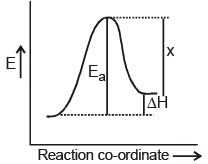Now when the products is converted back to reactant the energy of activation is x as shown in fig.

Evidently x = Ea - ΔH

= (77 - 72) = 5 kJ mol-1 Therefore, (C)

Problem : 2

In a certain reaction 10% of the reactant decomposes in the first hour, 20% is second hour, 30% in third hour and so on. What are the dimensions of rate constant.

(A) hour-1
(B) mol lit-1 sec-1
(C) lit mol-1 sec-1
(D) mol sec-1

Sol. If the amount of products formed which is 10%, 20% and 30% is plotted against time i.e., 1 hr, 2 hr and 3 hr respectively, it is a straight line passing through the origin.

Therefore, it is a zero order reaction where x = kt ⇒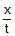= k

Therefore, dimensions of k = moles lit-1 sec-1

Therefore, (B)

Problem : 3

Two substances A(t½ = 5 mins) and B(t½ = 15 mins) are taken is such a way that initially [A] = 4[B]. The time after which the conentration of both will be equal is

(A) 5 min

(B) 15 min

(C) 20 min

(D) concentration can never be equal

Sol. t½ of A is 5 min

Therefore, in 15 mins it will become 1/8 of initial and t½ of B is 15 mins

Therefore, in 15 mins it will become ½ of initial

Therefore, ratio of [A] : [B] after 15 min is 4 : 1

But given [A] = 4[B]

Therefore, [A] = [B] after 15 min

Therefore, [B]

Problem : 4

The reaction A(g) 2B(g) → C(g) D(g) is an elementary process. In an experiment, the initial partial pressure of A & B are PA = 0.60 and PB = 0.80 atm. When Pc = 0.2 atm the rate of reaction relative to the initial rate is

(A) 1/48

(B) 1/24

(C) 9/16

(D) 1/6

Sol.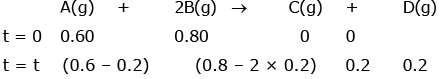(Rate)i = k[A] [B]2 = k[0.6][0.8]2

(Rate)t = k[0.4] [0.4]2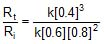=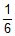Therefore, [D]

Problem : 5

For a hypothetical reaction A B → C D, the rate = k[A]-1/2[B]3/2. On doubling the concentration of A and B the rate will be

(A) 4 times

(B) 2 times

(C) 3 times

(D) none of these

Sol. k = k-1/2 3/2 = k3/2-1/2 = k = 1 = 2k

Therefore, [B]

Problem : 6

An organic compound A decomposes by following two parallel first order mechanisms :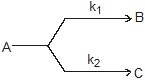;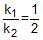, k1 = 0.693 hr -1

Select the correct statement(s)

(A) If three moles of A are completely decomposed then 2 moles of B and 1 mole of C will be formed.

(B) If three moles of A are completely decomposed then 1 moles of B and 2 mole of C will be formed.

(C) half life for the decomposition of A is 20 min

(D) half life for the decomposition of B is 0.33 min

Sol. BC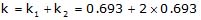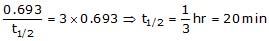Problem : 7

For any Ist  order gaseous reaction A → 2B Pressure devoloped after 20 min is 16.4 atm and after very long time is 20 atm. What is the total pressure developed after 10 min.

(A) 12

(B) 13

(C) 14

(D) 15

Sol. C

A(g)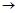2B(g)

t = 0 PO -

t = 10 PO - P 2P

t = 20 PO - P' 2P'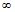- 2P

2PO = 20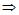PO = 10

16.4 = PO - P' 2P'

16.4 = 10 P'

P' = 6.4

for first order reaction at equal time conc/pr is in G. P.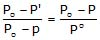,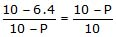3.6 × 10 = (10 - P)2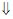10 - P = 6

P = 4

P10 min = PO P = 10 4 = 14 atm

Problem : 8

For any reaction, 2A → B, rate constant of reaction is 0.231 min-1. Time (in sec) when 25% of A will remain unreacted.

(A) 150

(B) 180

(C) 200

(D) 140

Sol.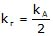,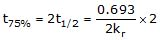= 3 mm

= 180 sec

Problem : 9

For any reaction A(g) → B(g), rate constant k = 8.21 × 10-2 atm/min at 300 K. If initial concentration of A is 2M then what is the half life (in hr.)?

(A) 5

(B) 6

(C) 7

(D) 8

Sol. A

Rate = k =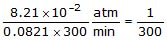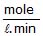t1/2 =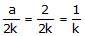= 300 min

= 5 hr.

Problem : 10

2A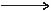B C

The mechanism of the above reaction given as

2A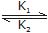X 2B (fast)

X B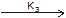C (slow)

E1 = Activation energy for K1

E2 = Activation energy for K

E3 = Activation energy for K3

Calculate Ereaction

(given : E1 = 10 kJ, E3 = 5 kJ, E2 = 12 kJ)

(A) 5

(B) 6

(C) 7

(D) 3

Sol. D

rate = K3 [x][B]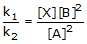, [X] =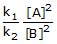rate = k3 .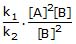, = k3 .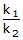.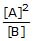Keff  =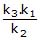, Etotal = E1 E3 - E2

= 3kJ

Offer running on EduRev: Apply code STAYHOME200 to get INR 200 off on our premium plan EduRev Infinity!

## Chemistry for JEE

198 videos|450 docs|370 tests

,

,

,

,

,

,

,

,

,

,

,

,

,

,

,

,

,

,

,

,

,

;